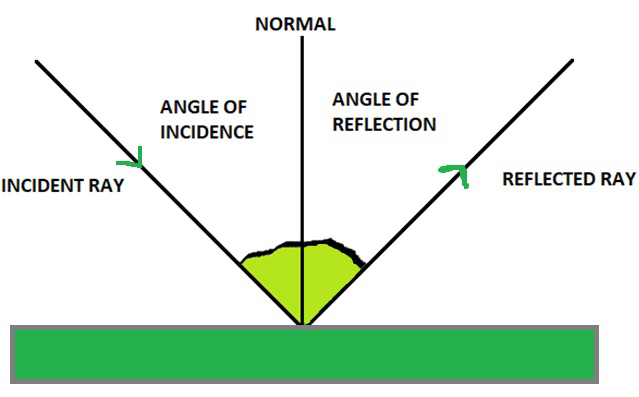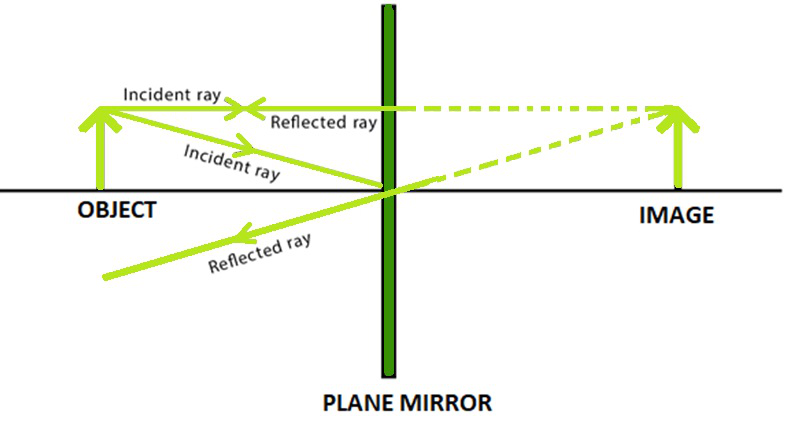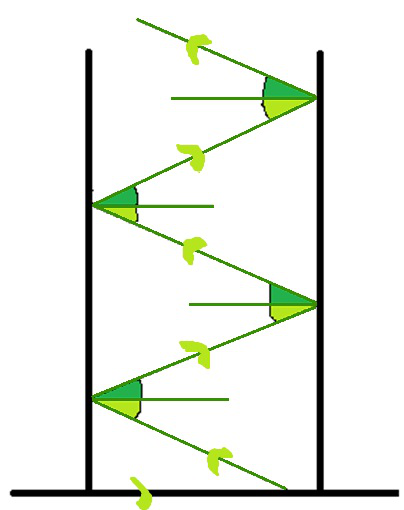# Multiple Reflections of Light

• Last Updated : 03 Mar, 2021

The process of bouncing back light rays which fall on the surface of an object, is called the reflection of light. A plane mirror reflects almost all the light that falls on it. In order to study and observe the reflection of light, we need an apparatus that can produce a thin beam of light. In other words, Reflection is the change in direction of a definite wave at a boundary between two different media, so that the wave moves back into the medium from which it came from. Some of the common examples include the reflection of light, sound, and water waves. In simpler words, Reflection of light is the phenomenon of bouncing back of light in the same medium on striking the surface of any distinct object.

Following the phenomenon of reflection two laws of reflection has been derived as stated below:

• The angle of incidence is always equal to the angle of reflection.
• The incident ray, the normal formed at the point of incidence, and the reflected ray, all lie in the same plane.Reflection from Mirror Surface

### Plane Mirror

A plane mirror refers to a mirror with a flat (planar) reflective surface. The light rays when striking a plane mirror, the angle of reflection always equals the angle of incidence. The angle of the incidence refers to the angle between the incident ray and the surface normal (an imaginary line perpendicular drawn to the surface). Similarly, the angle of reflection is the angle between the reflected ray and the normal at the point of incidence.

Concave and convex mirrors which come under spherical mirrors are also able to produce virtual images of objects similar to a plane mirror. But, the images formed by them are not always of the same size as the object like in the case of a plane mirror. The image by a convex mirror, the virtual image formed is always diminished in size, however in the case of a concave mirror when the object is placed somewhere between the focus and the pole of the mirror, an enlarged virtual image can be seen. However, when we need a virtual image of the same size a plane mirror is preferred over spherical mirrors.

Characteristics of the image formed by plane mirror:

• The image formed is exactly of the same size as the object.
• The position of the image beyond the mirror is the same as that of the object in front.
• The image formed is virtual.
• The plane mirror always forms a real image of the object.
• The image obtained in the plane mirror is always erect.
• The phenomenon of lateral inversion can be observed. Where the right appears to be left and vice versa.

Let us seek some examples to better understand that images can be reflected again by the surface of plane mirrors:

Have you ever seen a reflection of a scenic picture or your dirty laundry? The mirror always doubles back what it reflects so if we want to make sure that whatever we see in it is what we want to see. While speaking of the doubling, when we have two mirrors across from each other, they are streaming energy towards the back and forth between them.

We have sometimes observed that whenever we visit a barber, he focuses the mirror on our backside. This setup focuses the light straightway into our hair and helps the barber to cut the hair properly.

After the haircut the barber places a mirror at the back of ours so that we can see the image of our hair in the mirror in front, the hair cut can be seen very clearly in the mirror in front. In this, state the reflected light is reflected backward again from one mirror to another. When the two mirrors are put inclined at an angle to each other they can collectively form multiple images of the object in front.The above image shows the image formed by a plane mirror. As stated above the characteristics of the image formed by the plane mirror can be observed very clearly in the image. The size, distance, etc are all equal and a virtual, erect, and laterally inverted image is formed.

Reflected light can be reflected again applies up to the time when the light loses all its energy.

For example, a Kaleidoscope demonstrates a well-known example of this statement. In a kaleidoscope, two or more mirrors are placed at a certain angle to each other so that the reflective lights can reflect multiple times, and the object of which the image is to be seen is placed at one end of the mirrors. Due to repeated reflections, we can see multiple images which are very distinct from the object. Sometimes, in fair people use this trick to show their customers different images of their hand when they observe it under a kaleidoscope. People often use these images as an idea to create some new designs for their artwork, textile, or any other purpose.

### Multiple Images

Multiple images are the images that are formed whenever two or more than two mirrors are kept in front of each other and an object is placed between them. We can observe the different number of images if we go on increasing the number of mirrors placed at an inclination to each other.

To calculate the number of images that are formed we place the plane mirrors tilted at an angle to each other, using the formulawhere, N=number of images formedangle between the mirrors

The number of images also depends upon the position of the object.

If the object is asymmetric two cases can be seen:

• If the value ofis even then the number of images is given by the formula,• If the value ofis odd then the number of images is given by the formula,If the object is symmetric then only one case arises:

• Irrespective of whether the value ofis the number of images that can be calculated using the formula,The above diagram shows multiple images which are formed by a plane mirror. As stated above the number of images depends upon the angle between the mirrors, so it is mandatory to have some inclination between the two mirrors or more than two mirrors for the multiple images to be formed.

We can also observe this phenomenon in some museums where there are many different kinds of mirrors which give the different number of images according to their inclination.

Sometimes while visiting a barbershop we have seen that if they have a mirror placed on both sides we can see the numerous images if we look into any one of them. Apart from the designing point of view, it is also done so that after cutting the hair the barber can show the hair cut very easily to us by placing a mirror at the back of us.

### Sample Questions

Question 1: Calculate the number of images formed when the two plane mirrors are kept at right angles to each other.

To calculate the number of images formed we use the following formula,Given, θ = 90°

Putting the value in the formula we get,Therefore, N = 4 – 1

Therefore, N = 3

So the number of images formed is 3.

Question 2: Determine the number of images formed when the two plane mirrors are inclined at an angle of 60^{o}to each other.

To calculate the number of images formed we use the following formula,Given, θ = 60°

Putting the value in the formula we get,Therefore, N = 6 – 1

Therefore, N = 5

So the number of images formed is 5.

Question 3: Calculate the number of images formed by an asymmetric object if the angle between the mirrors is

Given, θ = 40°

Putting the value in the formula we get,which is odd and the object is asymmetric

Hence we will use,to calculate number of images,

Therefore, N = 9

So the number of images formed is 9.

Question 4: Find out the inclination between the two mirrors if we have 11 images formed.

To calculate we will use the following formula,Given, N=11Therefore, θ = 30°

Hence, the angle of inclination is 30°.

Question 5: Name a device that can show multiple images of the objects clearly with many patterns if the objects used are marbles of different colours.

Kaleidoscope can show multiple images of the marbles with many beautiful patterns.

Question 6: Write any two characteristics of the image formed by the plane mirrors?

The following are the two characteristics:

• Size of image is same as that of object.

• The image distance from the mirror is same as that of the object.

Question 7: How many laws of reflection are there? State them.